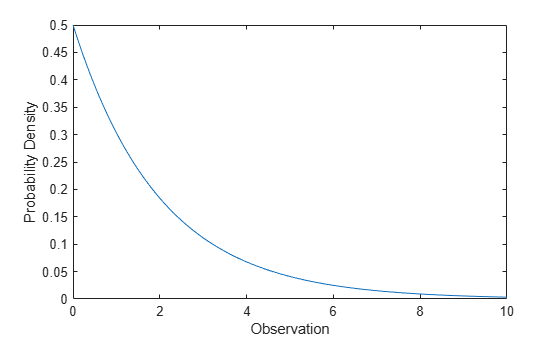Documentation

## Exponential Distribution

### Definition

The exponential pdf is

`$y=f\left(x|\mu \right)=\frac{1}{\mu }{e}^{\frac{-x}{\mu }}$`

### Background

Like the chi-square distribution, the exponential distribution is a special case of the gamma distribution (obtained by setting a = 1)

`$y=f\left(x|a,b\right)=\frac{1}{{b}^{a}\Gamma \left(a\right)}{x}^{a-1}{e}^{\frac{x}{b}}$`

where Γ( · ) is the Gamma function.

The exponential distribution is special because of its utility in modeling events that occur randomly over time. The main application area is in studies of lifetimes.

### Parameters

Suppose you are stress testing light bulbs and collecting data on their lifetimes. You assume that these lifetimes follow an exponential distribution. You want to know how long you can expect the average light bulb to last. Parameter estimation is the process of determining the parameters of the exponential distribution that fit this data best in some sense.

One popular criterion of goodness is to maximize the likelihood function. The likelihood has the same form as the exponential pdf above. But for the pdf, the parameters are known constants and the variable is x. The likelihood function reverses the roles of the variables. Here, the sample values (the x's) are already observed. So they are the fixed constants. The variables are the unknown parameters. MLE involves calculating the values of the parameters that give the highest likelihood given the particular set of data.

The function `expfit` returns the MLEs and confidence intervals for the parameters of the exponential distribution. Here is an example using random numbers from the exponential distribution with µ = 700.

```lifetimes = exprnd(700,100,1); [muhat, muci] = expfit(lifetimes)```
```muhat = 672.8207 muci = 547.4338 810.9437```

The MLE for parameter µ is 672, compared to the true value of 700. The 95% confidence interval for µ goes from 547 to 811, which includes the true value.

In the life tests you do not know the true value of µ so it is nice to have a confidence interval on the parameter to give a range of likely values.

### Examples

For exponentially distributed lifetimes, the probability that an item will survive an extra unit of time is independent of the current age of the item. The example shows a specific case of this special property.

```l = 10:10:60; lpd = l+0.1; deltap = (expcdf(lpd,50)-expcdf(l,50))./(1-expcdf(l,50))```
```deltap = 0.0020 0.0020 0.0020 0.0020 0.0020 0.0020```

#### Compute the Exponential Distribution pdf

Compute the pdf of an exponential distribution with parameter `mu = 2`.

```x = 0:0.1:10; y = exppdf(x,2);```

Plot the pdf.

```figure; plot(x,y)```